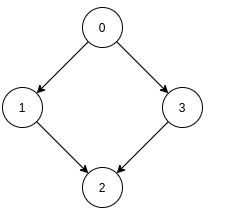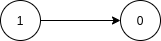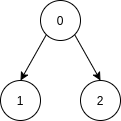New update is available. Click here to update.

# Topological Sort

Contributed by
Vishal Modani
Last Updated: 23 Feb, 2023
Medium0/80
Avg time to solve 30 mins
Success Rate 70 %Share77 upvotes

## Problem Statement

#### For example, Consider the DAG shown in the picture.#### Note that there are multiple topological sortings possible for a DAG. For the graph given above one another topological sorting is: {0, 3, 1, 2}

##### Note:
``````1. It is guaranteed that the given graph is DAG.
2. There will be no multiple edges and self-loops in the given DAG.
3. There can be multiple correct solutions, you can find any one of them.
4. Don’t print anything, just return an array representing the topological sort of the vertices of the given DAG.
``````
Detailed explanation ( Input/output format, Notes, Images )##### Constraints:
``````1 <= T <= 50
1 <= V <= 10^4
0 <= E <= 10^4
0 <= u, v < V

Where ‘T’ is the total number of test cases, ‘V’ is the number of vertices, ‘E’ is the number of edges, and ‘u’ and ‘v’ both represent the vertex of a given graph.

Time limit: 2 sec
``````
##### Sample Input 1:
``````2
2 1
1 0
3 2
0 1
0 2
``````
##### Sample Output 1:
``````1 0
0 2 1
``````
##### Explanation of Sample Input 1:
``````Test case 1:
The number of vertices ‘V’ = 2 and number of edges ‘E’ = 1.
The graph is shown in the picture:
````````````The topological sorting of this graph should be {1, 0}  as there is a directed edge from vertex 1 to vertex 0, thus 1 should come before 0 according to the given definition of topological sorting.

Test case 2:
The number of vertices ‘V’ = 3 and number of edges ‘E’ = 2.
The graph is shown in the picture:
````````````As there are two directed edges starting from 0, so 0 should come before 1 and 2 in topological sorting.
Thus the topological sorting of this graph should be {0, 2, 1} or {0, 1, 2}
``````
##### Sample Input 2:
``````2
1 0
4 4
0 1
0 3
1 2
3 2
``````
##### Sample Output 2:
``````0
0 1 3 2
``````
##### Explanation of Sample Input 2:
``````Test case 1:
There is only a single vertex in the graph that is 0, so its topological sort will be {0}.

Test case 2:
See problem statement for its explanation
``````AutoConsole